1597449780

# Statistical Measures of Central Tendency

## Introduction

In statistics, measures of central tendency are a set of “middle” values representative of the data points. Central tendency describes the distribution of data focusing on the central location around which all other data are clustered. It is the opposite of dispersion that measures how far the observations are scattered with respect to the central value.

As we will see below, central tendency is an elementary statistical concept, yet a widely used one. Among the measures of central tendency mean, median and mode are most frequently cited and used. Below we will see why they are important in the field of data science and analytics.

## 1. Arithmetic Mean

Mean is the average of some data points. It is the simplest measure of central tendency that takes the sum of the observations and divides the sum by the number of observations.

In mathematical notation arithmetic mean is expressed as: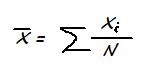Where xi are individual observations and N is the number of observations

In a more practical example, if wages of 3 restaurant employees are \$12, \$14 and \$15 per hour, then the average wage is \$13.6 per hour. Simple as that.

#statistics #machine-learning #median #data-science #mean #deep learning

## Buddha Community1593039000

## What is Central Tendency?

Central Tendency is the measure of very basic but very useful statistical functions that represents a central point or typical value of the dataset. It help’s in indicating the point value where the most value in the distribution falls referring to the central location of the distribution. The most common central tendency methods used for the analysis of numerical data are mean, median, and mode.

## Mean

The mean is the most common and well-known method for measuring central tendency and can be used to handle both discrete and continuous data. We can calculate mean as the sum of all the values in the dataset divided by the number of values in the dataset and is denoted as ‘µ’.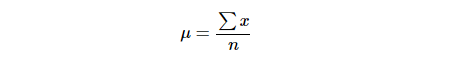Mean is not often one of the actual values that you have observed in your data set but it is one of the most important properties as it minimizes the error to predict the value in any dataset. The reason behind having the lowest error is because it includes every value in your data set as part of the calculation. In addition, the mean is the only measure of central tendency where the sum of the deviations of each value from the mean is always zero.

In the below image we can see the histogram for an array of values and then calculated the mean by summing all the values on the x-axis and just dividing by the number of values i.e 12.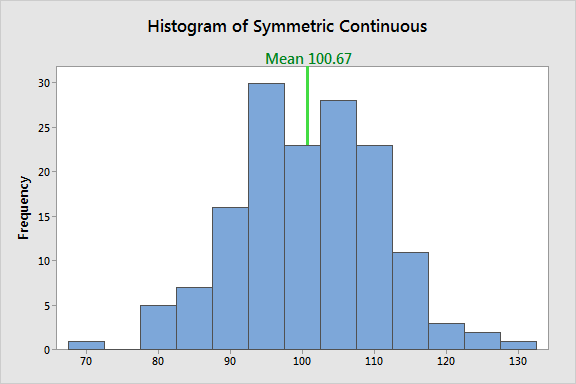However, the disadvantage of using the mean is that it is particularly susceptible to the influence of outliers. Outliners are the value that is very unusual as compared to the rest of the data, like making a particular value being very small or very large as compared to the rest. Focusing the case when our data is skewed or we can say that when the data is perfectly normal, the mean, median, and mode are identical. In this case, mean lose its ability to provide the best central location for the data because the skewed data is dragging it away from the typical value.

The below histogram shows the image with the skewed dataset and hence all the three mean median and mode will be approx equal to each other.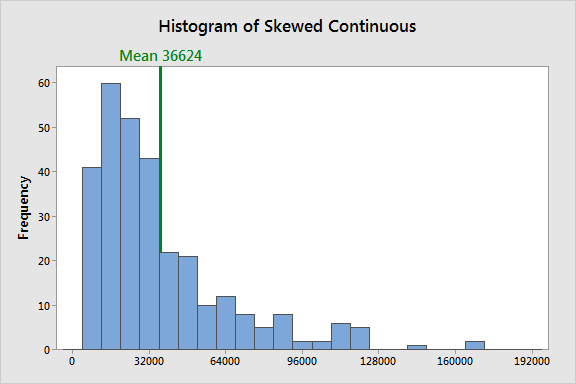## Median

Median is the middle value of your observation when the values in the dataset are ordered from the smallest to the largest. If the number of values in the dataset is an odd number then the middle value is the median. But if you have odd number values in the dataset then in order to find median we just take the average of the two middle values.

The below histogram shows the relationship between the mean and mode if we have symmetric data.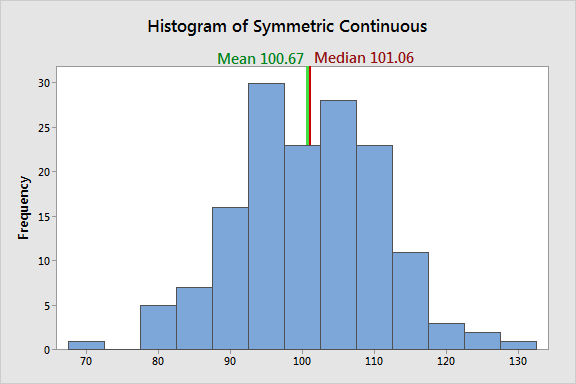#statistics #data-analysis #mean-median-mode #data-science #central-tendency1594267320

## Statistics: Central Tendency

In our quest to summarize data, either via data tables or visual effects, we wanted to represent the entirety of the data. However, often we have wished there was a single point that was representative of the data at hand. Using any extreme value in the data series would only explain one end of the series. So, it may be useful to use a central value with some observations larger or smaller than it. Such a measure is called central tendency. However, does a central point tell the entire story? You know some values are larger or smaller than the central tendency value, however it does not talk about the spread or heterogeneity in the data. This is what dispersion measures. The two concepts form a foundation for many advanced statistical concepts.

The aim of this article is to describe the different measures of central tendency and also introduce a bit of simple python coding to explain how to see them in practice. I’ll attempt to share some knowledge on dispersion in a follow up article.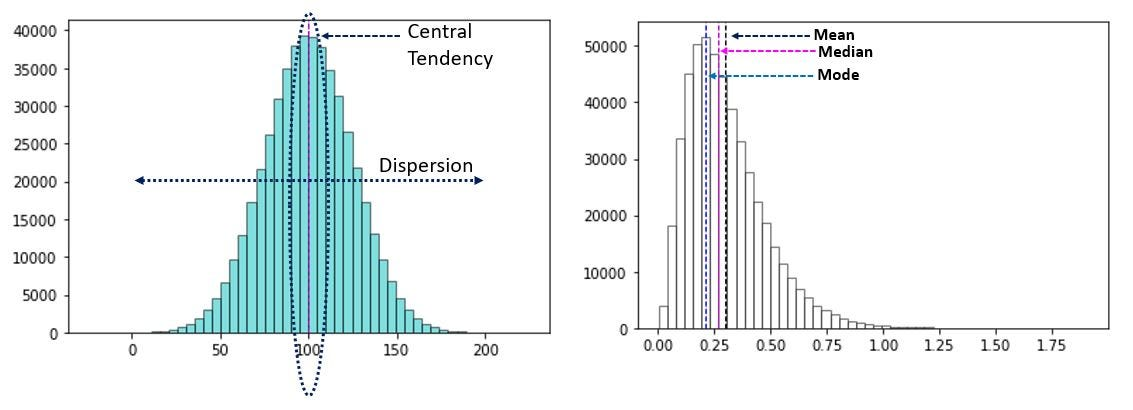Fig 1: Central Tendency & Dispersion of plotted data in images

# Central Tendency

Different measures of central tendency can be easily demonstrated by the below chart: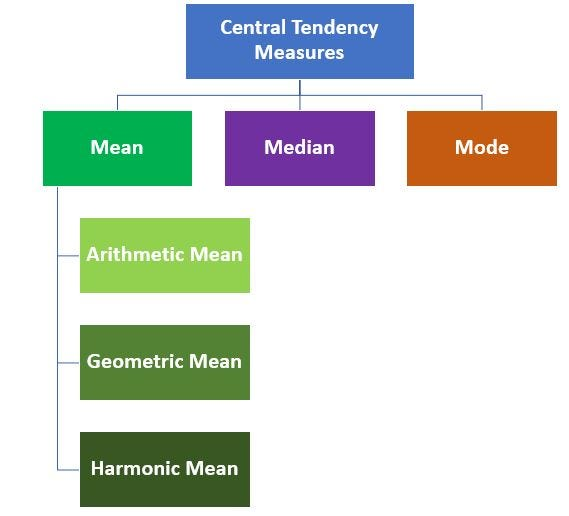Fig 2: Different Measures of Central Tendency

As evident from the image, central tendency can be classified into 3 classes — mean, median and mode. Before we get into the details of each, it is worth taking a look at the requisite properties of an ideal measure of central tendency as defined by Prof. Udny Yule:

• The measure needs to be based on all observations in the data,
• based on all observations,
• affected as little as possible by sampling fluctuations
• rigidly defined, easy to calculate, readily comprehensible and
• there is possibility to do further mathematical work

With this in mind, let us take a look at different measures of Central Tendency below. Also, 2 noteworthy concepts to note here are population and sample. Population is the collection of all possible observations in a data set while sample is a subset drawn from the population using different techniques.

## Mean

The mean is calculated by various mathematical operations on all observations in a data series:

• **Arithmetic Mean: **One of the easiest to calculate measures, arithmetic mean is defined as the sum of all observations in a data series divided by the count of all observations in that series. Mathematically,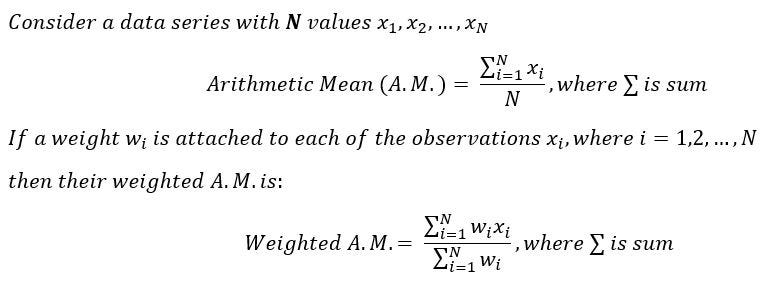One interesting property of A.M. to note here is that if each observation in a series is increased or decreased by the same constant, the A.M. of the new series would also increase or decrease by the same constant.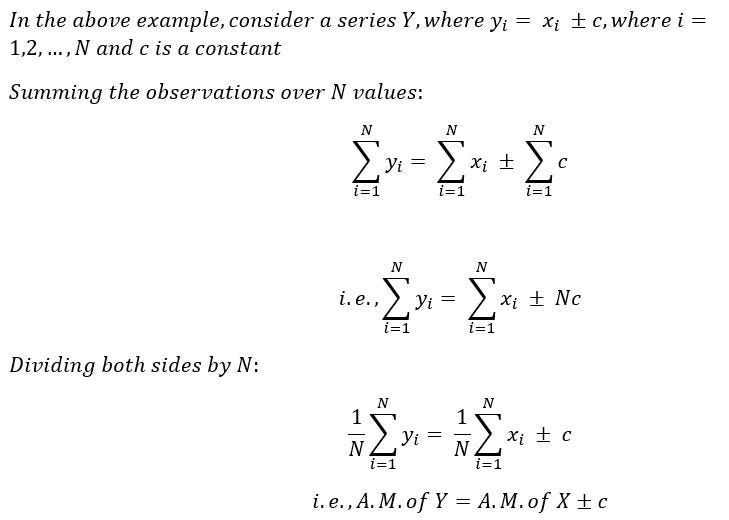• Geometric mean: Geometric Mean is defined as the n-th root of the product of n observations in a data series. Mathematically,

Image for post

If the calculations can be taken a bit further, it is very easy to see that usage of logarithms can make the calculations easier.

#statistics #statistical-analysis #data analysis1593564878

## Levels of Measurements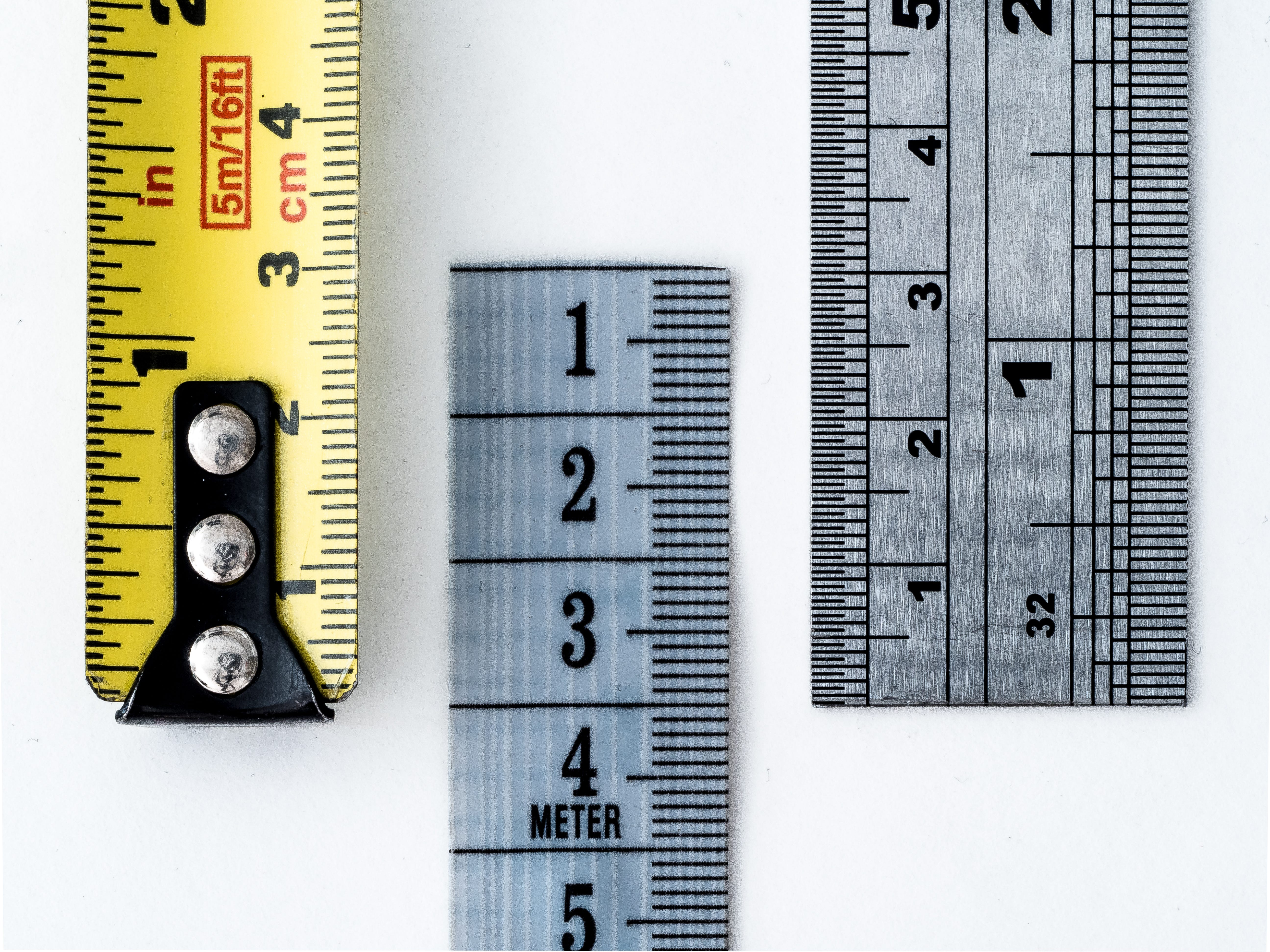Photo by William Warby on Unsplash

Measurement is the process of assigning numbers to quantities (variables). The process is so familiar that perhaps we often overlook its fundamental characteristics. A single measure of some attribute (for example, weight) of sample is called statistic. These attributes have inherent properties too that are similar to numbers that we assign to them during measurement. When we assign numbers to attributes (i.e., during measurement), we can do so poorly, in which case the properties of the numbers to not correspond to the properties of the attributes. In such a case, we achieve only a “low level of measurement” (in other words, low accuracy). Remember that in the earlier module we have seen that the term accuracy refers to the absolute difference between measurement and real value. On the other hand, if the properties of our assigned numbers correspond properly to those of the assigned attributes, we achieve a high level of measurement (that is, high accuracy).

American statistician Stanley Smith Stevens is credited with introducing various levels of measurements. Stevens (1946) said: “All measurements in science are conducted using four different types of scales nominal, ordinal, interval and ratio”. These levels are arranged in ascending order of increasing accuracy. That is, nominal level is lowest in accuracy, while ratio level is highest in accuracy. For the ensuing discussion, the following example is used. Six athletes try out for a sprinter’s position in CUPB Biologists’ Race. They all run a 100-meter dash, and are timed by several coaches each using a different stopwatch (U through Z). Only the stopwatch U captures the true time, stopwatches V through Z are erroneous, but at different levels of measurement. Readings obtained after the sprint is given in Table.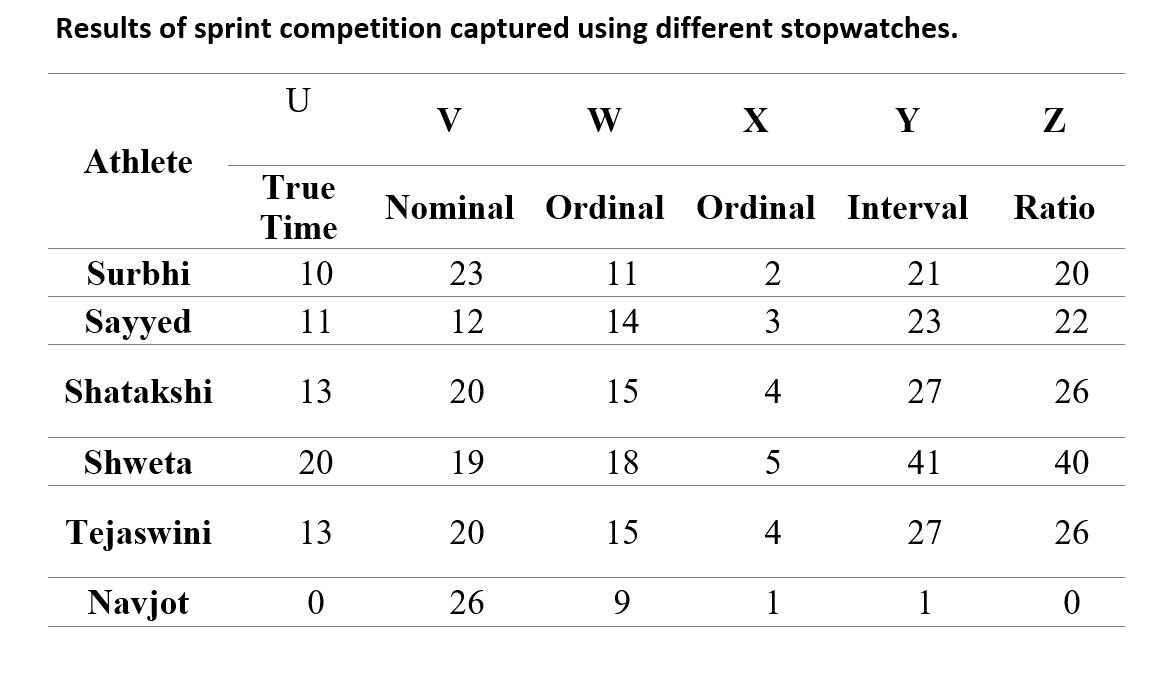# Nominal level of measurement

Nominal scale captures only equivalence (same or different) and set membership. These sets are commonly called categories, or labels. Consider the results of sprint competition, Table 1. Watch V is virtually useless, but it has captured a basic property of the running times. Namely, two values given by the watch are the same if and only if two actual times are the same. For example, participants Shatakshi and Tejaswini took same time in the race (13s), and as per the readings of stopwatch V, this basic property remains same (20s each). By looking at the results from stopwatch V, it is cogent to conclude that ‘Shatakshi and Tejaswini took same time in the race’. This attribute is called equivalency. We can conclude that watch V has achieved only a nominal level of measurement. Variables assessed on a nominal scale are called categorical variables. Examples include first names, gender, race, religion, nationality, taxonomic ranks, parts of speech, expired vs non expired goods, patient vs. healthy, rock types etc. Correlating two nominal categories is very difficult, because any relationships that occur are usually deemed to be spurious, and thus unimportant. For example, trying to figure out how many people from Assam have first names starting with the letter ‘A’ would be a fairly arbitrary, random exercise.

# Ordinal level of measurement

Ordinal scale captures rank-ordering attribute, in addition to all attributes captured by nominal level. Consider the results of sprint competition, Table 1. Ascending order of time taken by the participants as revealed by the true time are (respective ranks in parentheses): Navjot (1), Surbhi (2), Sayyed (3), Shatakshi and Tejaswini (4 each), and Shweta (5). Besides capturing the same-difference property of nominal level, stopwatches W and X have captured the correct ordering of race outcome. We say that the stopwatches W and X have achieved an ordinal level of measurement. Rank-ordering data simply puts the data on an ordinal scale. Examples at this level of measurement include IQ Scores, Academic Scores (marks), Percentiles and so on. Rank ordering (ordinal measurement) is possible with a number of subjective measurement surveys. For example, a questionnaire survey for the public perception of evolution in India included the participants to choose an appropriate response ‘completely agree’, ‘mostly agree’, ‘mostly disagree’, ‘completely disagree’ when measuring their agreement to the statement “men evolved from earlier animals”.

#measurement #data-analysis #data #statistical-analysis #statistics #data analysis1597449780

## Introduction

In statistics, measures of central tendency are a set of “middle” values representative of the data points. Central tendency describes the distribution of data focusing on the central location around which all other data are clustered. It is the opposite of dispersion that measures how far the observations are scattered with respect to the central value.

As we will see below, central tendency is an elementary statistical concept, yet a widely used one. Among the measures of central tendency mean, median and mode are most frequently cited and used. Below we will see why they are important in the field of data science and analytics.

## 1. Arithmetic Mean

Mean is the average of some data points. It is the simplest measure of central tendency that takes the sum of the observations and divides the sum by the number of observations.

In mathematical notation arithmetic mean is expressed as:Where xi are individual observations and N is the number of observations

In a more practical example, if wages of 3 restaurant employees are \$12, \$14 and \$15 per hour, then the average wage is \$13.6 per hour. Simple as that.

#statistics #machine-learning #median #data-science #mean #deep learning1595096220

## Factors That Can Contribute to the Faulty Statistical Inference

Hypothesis testing is a procedure where researchers make a precise statement based on their findings or data. Then, they collect evidence to falsify that precise statement or claim. This precise statement or claim is called the null hypothesis. If the evidence is strong to falsify the null hypothesis, we can reject the null hypothesis and adapt the alternative hypothesis. This is the basic idea of hypothesis testing.

## Error Types in Statistical Testing

There are two distinct types of errors that can occur in formal hypothesis testing. They are:

Type I: Type I error occurs when the null hypothesis is true but the hypothesis testing results show the evidence to reject it. This is called a false positive.

Type II: Type II error occurs when the null hypothesis is not true but it is not rejected in hypothesis testing.

Most hypothesis testing procedure performs well controlling type I error (at 5%) in ideal conditions. That may give a false idea that there is only a 5% probability that the reported findings are wrong. But it’s not that simple. The probability can be much higher than 5%.

## Normality of the Data

The normality of the data is an issue that can break down a statistical test. If the dataset is small, the normality of the data is very important for some statistical processes such as confidence interval or p-test. But if the data is large enough, normality does not have a significant impact.

## Correlation

If the variables in the dataset are correlated with each other, that may result in poor statistical inference. Look at this picture below: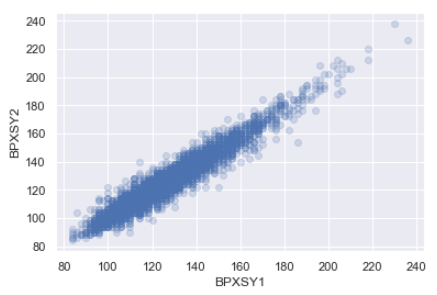In this graph, two variables seem to have a strong correlation. Or, if a series of data is observed as a sequence, that means values are correlated with its neighbors, and there may have some clustering or autocorrelation in the data. This kind of behavior in the dataset can adversely impact the statistical tests.

## Correlation and Causation

This is especially important when interpreting the result of a statistical test. “Correlation does not mean causation”. Here is an example. Suppose, you have study data that shows, more people who do not have college education believe that women should get paid less than men in the workplace. You may have conducted a good hypothesis testing and prove that. But care must be taken on what conclusion is drawn from this. Probably, there is a correlation between college education and the belief that ‘women should get paid less’. But it is not fair to say that not having a college degree is the cause of such belief. This is a correlation but not a direct cause ad effect relationship.

A more clear example can be provided from medical data. Studies showed that people with fewer cavities are less likely to get heart disease. You may have enough data to statistically prove that but you actually cannot say that the dental cavity causes heart disease. There is no medical theory like that.

#statistical-analysis #statistics #statistical-inference #math #data analysis